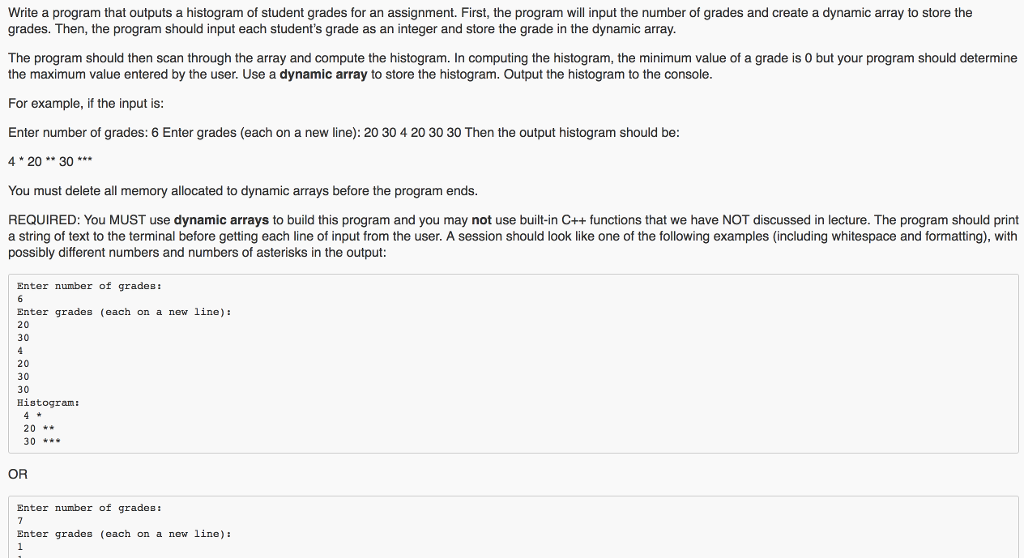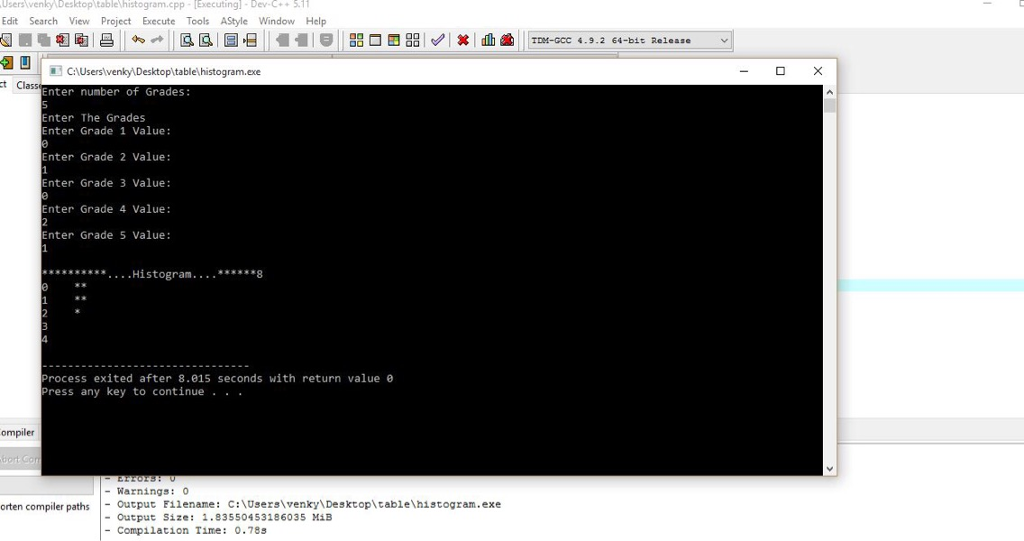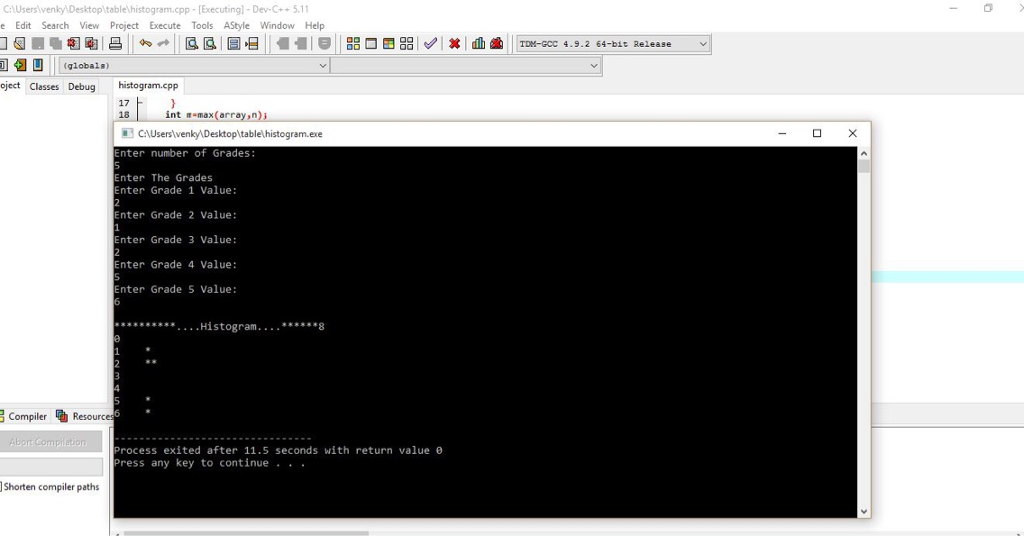# Answered! Write a program that outputs a histogram of student grades for an assignment. First, the program will input the…In C++, Write a program that outputs a histogram of student grades for an assignment. First, the program will input the number of grades and create a dynamic array to store the grades. Then, the program should input each student’s grade as an integer and store the grade in the dynamic array. The program should then scan through the array and compute the histogram. In computing the histogram, the minimum value of a grade is 0 but your program should determine the maximum value entered by the user. Use a dynamic array to store the histogram. Output the histogram to the console. For example, if the input is: Enter number of grades: 6 Enter grades (each on a new line): 20 30 4 20 30 30 Then the output histogram should be: 4 * 20 ** 30 *** You must delete all memory allocated to dynamic arrays before the program ends. REQUIRED: You MUST use dynamic arrays to build this program and you may not use built-in C++ functions that we have NOT discussed in lecture. The program should print a string of text to the terminal before getting each line of input from the user. A session should look like one of the following examples (including whitespace and formatting), with possibly different numbers and numbers of asterisks in the output: Enter number of grades: 6 Enter grades (each on a new line): 20 30 4 20 30 30 Histogram: 4 * 20 ** 30 *** OR Enter number of grades: 7 Enter grades (each on a new line): 1 1 100 100 100 99 50 Histogram: 1 ** 50 * 99 * 100 *** The strings printed by the program should include a newline at the end, but no other trailing whitespace (whitespace at the end of the line). The scores should be right-justified with width 3 (there should be two spaces before a one-digit number, and one space before a two-digit number). So you can assume that the “grades” inputted will not be composed of more than 3 digits. Hint for formatting: remember setw.

Don't use plagiarized sources. Get Your Custom Essay on
Answered! Write a program that outputs a histogram of student grades for an assignment. First, the program will input the…
GET AN ESSAY WRITTEN FOR YOU FROM AS LOW AS \$13/PAGE#include<iostream>

#include<conio.h>
#include<iomanip>
using namespace std;
int max(int *,int n);
int main()
{
int n,size;
cin>>n;
int *array= new int[n];
for(int i=0;i<n;i++)
{
cin>>array[i];
}
int m=max(array,n);
if(m>n)
size=m+1;
else
size=n;
int array2[size];
for(int i=0;i<size;i++)
{
array2[i]=0;
}
for(int i=0;i<n;i++)
{
array2[array[i]]++;
}
cout<<“n**********….Histogram….******8n”;
for(int i=0;i<size;i++)
{
cout<<left;
cout<<setw(5)<<i;
//cout<<setw(5)<<array2[i];
for(int j=1;j<=array2[i];j++)
{
cout<<“*”;
}
cout<<“n”;
}
return 0;
}
int max(int arr[],int n)
{
int large=arr;
for(int i=0;i<n;i++)
if(large<arr[i])
{
large=arr[i];
}
return large;
}